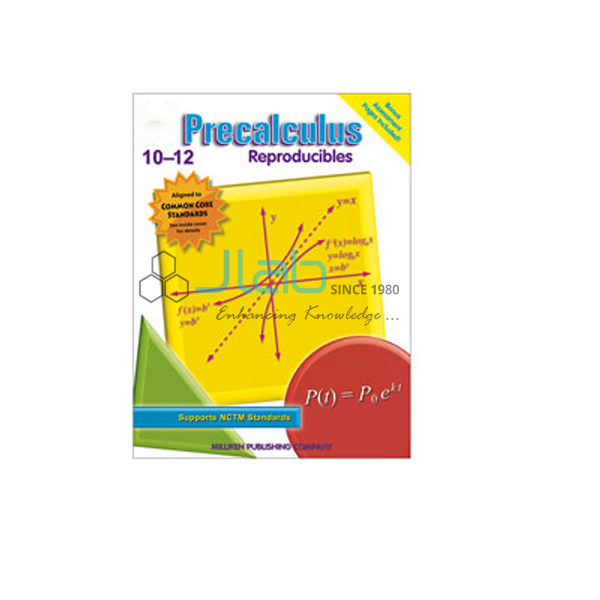# Precalculus Reproducible BookProduct Code : JL-MLI-9751

Precalculus Reproducible Book
Give students all the essential tools for a solid introduction to precalculus.
The skills required are introduced along with a variety of rules, theorems, processes and easy-to-follow examples.
Students use rules and theorems in a variety of stimulating activities to build a solid understanding of major concepts.
Games and puzzles use answers to practice problems to reinforce learning and make math fun.